# WA Multiscale Edge Detection VI

LabVIEW 2014 Advanced Signal Processing Toolkit Help

Edition Date: June 2014

Part Number: 372656C-01

»View Product InfoDownload Help (Windows Only)

Owning Palette: Feature Extraction VIs

Uses the multiresolution wavelet analysis to extract the edges of a signal.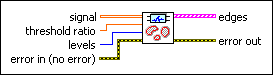signal specifies the 2D input signal.threshold ratio specifies the relative threshold to use to reject small peaks. threshold ratio is defined in the range [0, 1], where 0 and 1 correspond to the minimum and maximum values of the coefficients, respectively. The smaller the value of threshold ratio, the more edges this VI detects.levels specifies the number of levels in the discrete wavelet analysis. levels must be a positive integer no greater than log2(Ls), where Ls is the length of the 1D signal or the minimum dimensional size of the 2D signal. Otherwise, you can set the value to –1, which indicates that this VI sets levels as the largest integer no greater than log2(Ls). The default is –1.error in describes error conditions that occur before this node runs. This input provides standard error in functionality.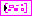edges returns the detected edges at different levels. The ith element of edges contains the edges of signal at the (i+1)th level. The edges at smaller levels reveal the details of the image, and the edges at larger levels provide the global and large-scale edges of the image, for example, the contour of an image.edge returns the edge of a level. edge is a binary image. 1 indicates that this VI detects an edge. You can display edge on an intensity graph or with the WA Gray Scale Image control.error out contains error information. This output provides standard error out functionality.

## WA Multiscale Edge Detection Details

Usually, the modulus-maxima of the detail coefficients of signal corresponds to the edge of signal. This VI completes the following steps to implement the multi-scale edge detection.

1. Performs the undecimated wavelet transform with wavelet bior3_1 on the rows and the columns, respectively, at each level. Obtains the detail coefficients along the row direction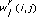and the column direction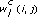. (i, j) denotes the row and column index of the matrix, respectively.
2. Computes a new matrix Rl with the formula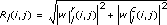.
3. Finds the local maxima of Rl as the edge points at level l.